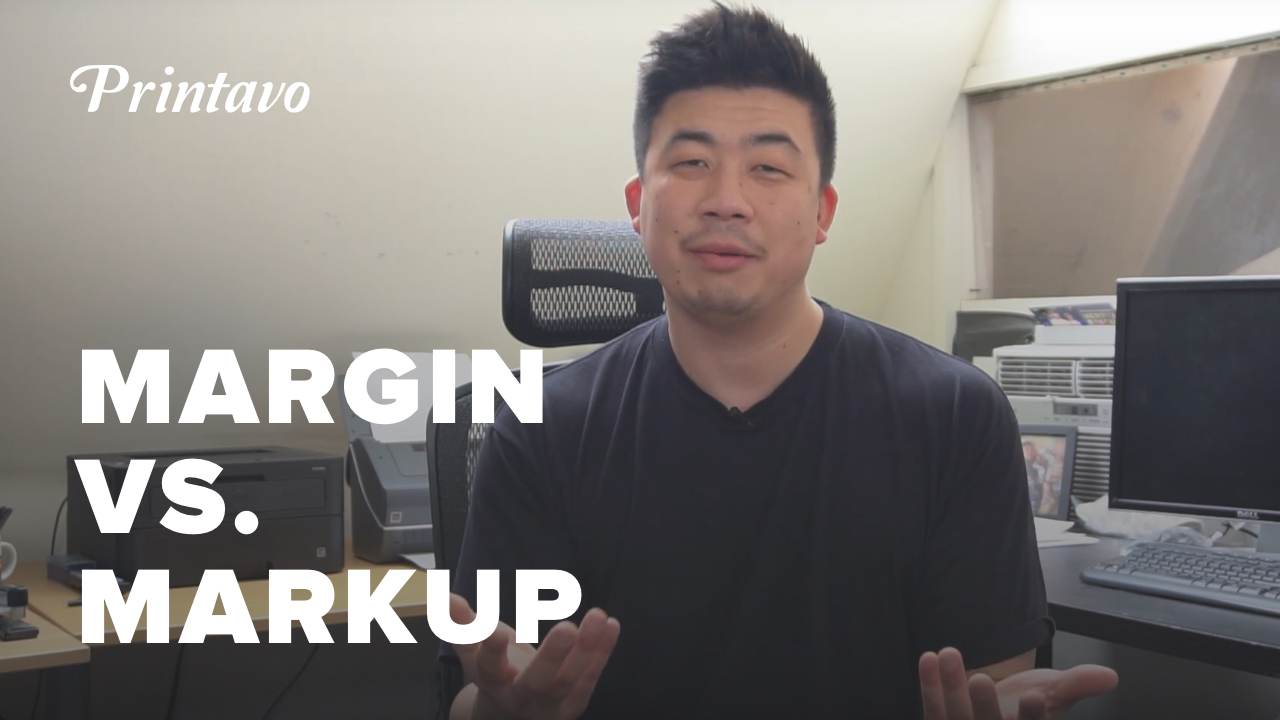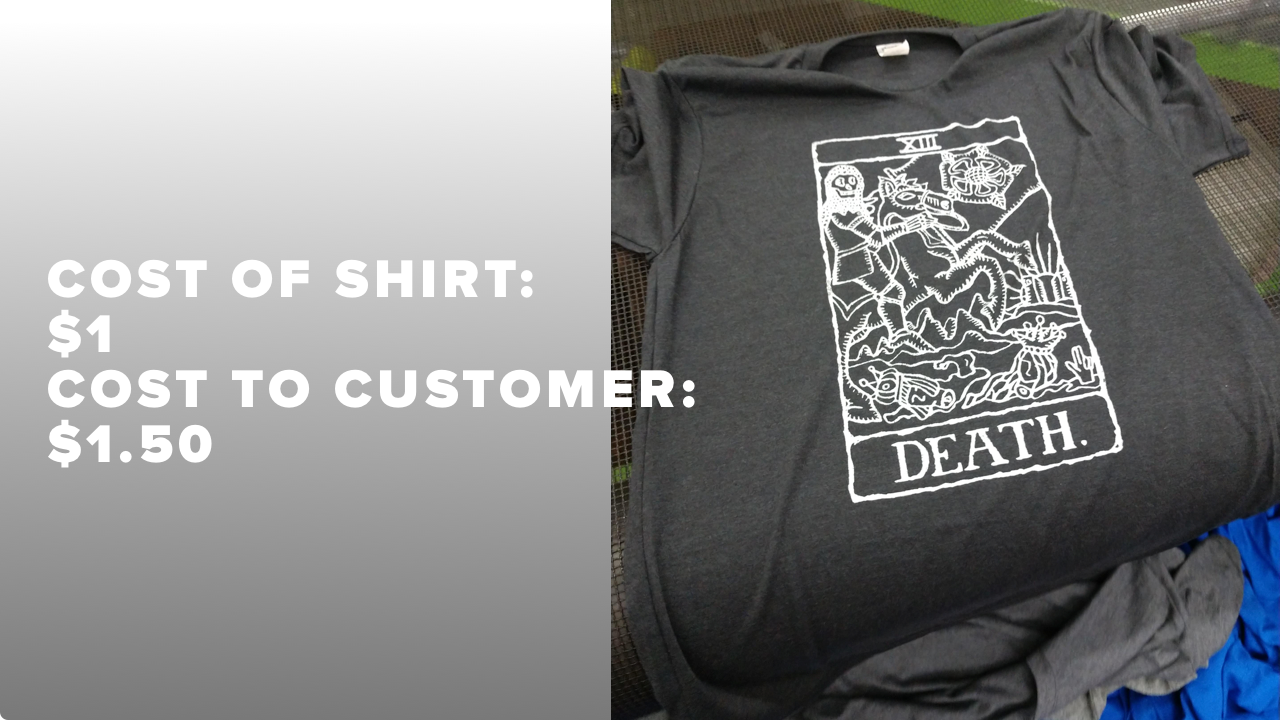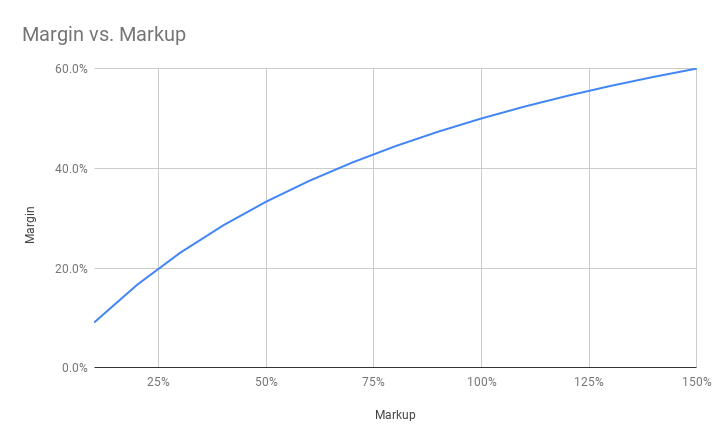# Markup vs. Margin: They're Not The Same for Your Pricing!About the author: Mike Chong owns Merch Monster in Oakland, CA. His primary focus is providing high quality custom apparel and merchandise for businesses across the US. He regularly produces video content for Printavo, which you can find in our Tips & Tutorials playlist on the Printavo YouTube channel.

Small business owners confuse markup and margin all the time. Mike Chong from Merch Monster in Oakland, CA wants to clear things up for everyone.

So let's dig in and show you the difference between your markup and your margin.

## Why you should understand the difference

There are two big reasons to have a firm grasp on your markup and your margin.

1. Predictability. Margin is used to calculate your future financials. Understanding your margin allows you to understand the health and sustainability of your business.
2. Pricing. Markup is the simplest way to manipulate your pricing. Understanding how your markup relates to your margin lets you control the profitability of each job.

Here's why these matter: predictable profits are the only route to long-term sustainability. If you don't know your margin and how it relates to your markup, you may miscalculate your future financials.

With a firm grasp of these basic concepts, you can chart a course toward profitability, adjust your prices to get there, and have a better vision for where you are in relation to your revenue and profitability goals.

## Markup versus marginLet's give you concrete (but simplified) examples to demonstrate markup versus margin.

You purchase a t-shirt from a vendor at \$1.00. For simplicity, we will call this the cost of goods.

Then you charge your customer \$1.50 for the shirt. For simplicity, we will call this the revenue.

On this sale, your gross profits are assumed to be \$0.50.

### Calculating markup

To calculate your markup, we use a very simple formula:

(Gross profits / Cost of goods) * 100 = Markup %

Plugging in our specific example, this is what we get:

(Gross profits (\$0.50) / Cost of goods (\$1.00)) * 100 = 50% markup

### Calculating margin

To calculate your margin, we use another simple formula:

(Gross profits / Revenue) * 100 = Margin %

Plugging in the values from our example, this is what we get:

(Gross profits (\$0.50) / Revenue (\$1.50)) * 100 = 33.3% margin

In plain English: Markup is the percentage you charge customers above the cost of the shirt you're printing on. Margin is the ratio of your profit to the price you charge your customers.

Below, you can see how markup percentages compare to margin percentages.Notice that markup does not have a 1:1 correlation with margin.

As you can see, raising your markup by a certain percentage does not raise your margin by an equivalent percentage. This is the key insight – and point of confusion – for many business owners.

## Calculating prices from a desired margin

So, let's say you want to achieve a specific percentage margin on a job you're printing. This is quite an easy calculation to make, and you only need a couple of pieces of information:

• The cost of the goods sold.
• It's recommended that you include production costs in this number.

Let's go back to our simplified example: a \$1 shirt sold for \$1.50. The margin is only 33.3%, but we actually want to achieve a 50% margin. How would you calculate the amount to raise your price by?Here's how this is done:

Cost of goods / 1 - Desired margin = Target price

Since we know the cost of goods (\$1) and our desired margin (50%), we can easily calculate our new price. Note: we must convert our percentage (50%) to a decimal (0.5) for this formula to work.

Cost of goods (\$1) / 1 - Desired margin (0.5) = Target price (\$2)

This gives us a new gross profit of \$1 and a new revenue of \$2.

Now, let's use our earlier formulas to ensure this calculation achieves what we want it to. First, we'll check that the margin is correct:

(New gross profit (\$1) / New revenue (\$2)) * 100 = 50% margin

Then we'll quickly check to see what our new markup percentage is:

(New gross profit (\$1) / Cost of goods (\$1)) * 100 = 100% markup

In other words, our markup doubled (from 50% to 100%) while our margin only increased by 66% (from 33% to 50%).

## Conclusion

Don't want to use a calculation? Download the Cost Margin Calculator app to get an intuitive sense of your markup and margin.

Got a tip for how you handle your margins? Let us know. In the meantime, check out Mike's other tips and tricks:

Looking to put profit first? Join us at PrintHustlers Conf 2019, July 12-13 in downtown Chicago. We've got Ryonet's Ryan Moor, industry consultant and expert Mark Coudray, and Profit First author Mike MichalowiczThere are only a handful of spaces remaining – so get your ticket today!